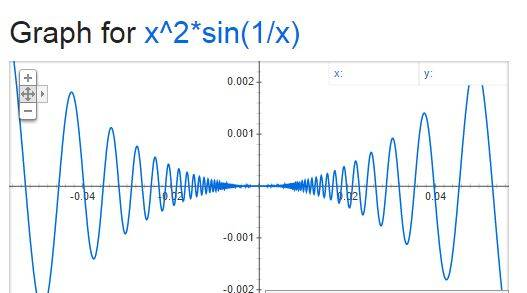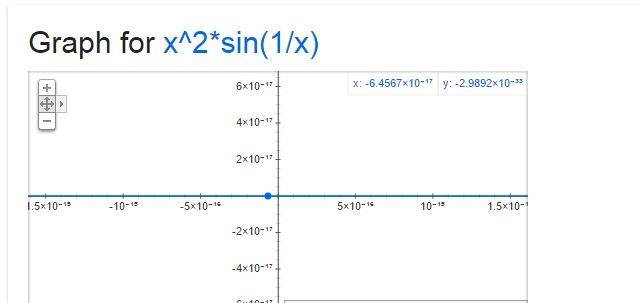# Solving tricky limit (involving defined integral and sine)

• lep11
Look at post #11.)Then you should remember that the derivative formula is for a point x, not for a value of x. So if you want to know the derivative at x=0, you should calculate ##\displaystyle \lim_{x \to 0} \frac{f(x)-f(0)}{x-0}##, not ##\displaystyle \frac{f(0)-f(0)}{0-0}##.The limit you get (using L'Hopital) is ##\displaystyle \lim_{x \to 0} 2x \sin(1/x)-\cos(1/x)##.

## Homework Statement

Calculate ##\lim_{x\to 1} \frac{1}{x-1} \int_{1}^{f(x)} sin(\pi t^2) dt##. f is differentiable in the neighbourhood of point ##x=1## and ##f(1)=1##.

## Homework Equations

If ##f## is continuous on a closed interval ##[a,b]##, then there exists ##ξ∈]a,b[## such that

##\int_{a}^{b} f(x)dx=f(ξ)(b-a)##.
( First Mean Value Theorem for Integrals)

## The Attempt at a Solution

I think the trick is to use the First Mean Value Theorem for Integrals. Obviously ##\sin(\pi t^2)## is continuous everywhere and so for a fixed ##x≠1##; ##\frac{1}{x-1} \int_{1}^{x} sin(\pi t^2) dt=\frac{1}{x-1}(x-1)\sin(\pi ξ^2)=\sin(\pi ξ^2)##. Therefore ##\lim_{x\to 1} \frac{1}{x-1} \int_{1}^{x} sin(\pi t^2) dt=\lim_{x\to 1} sin(\pi ξ^2)=0 ##

Last edited:
lep11 said:

## Homework Statement

Calculate ##\lim_{x\to 1} \frac{1}{x-1} \int_{1}^{f(x)} sin(\pi t^2) dt##. f is differentiable in the neighbourhood of point ##x=1## and ##f(1)=1##.

## Homework Equations

If ##f## is continuous on a closed interval ##[a,b]##, then there exists ##ξ∈]a,b[## such that

##\int_{a}^{b} f(x)dx=f(ξ)(b-a)##.
( First Mean Value Theorem for Integrals)

## The Attempt at a Solution

I am supposed to use the First Mean Value Theorem for Integrals. Obviously ##\sin(\pi t^2)## is continuous everywhere.
The hint is right there: "I am supposed to use the First Mean Value Theorem for Integrals".

Apply this to ##\displaystyle \frac{1}{x-1} \int_{1}^{f(x)} \sin(\pi t^2) dt## for a fixed ##x \neq 1##, and remember that ##ξ∈]1,f(x)[## (or ##ξ∈]f(x),1[## to be precise).

Then take the limit.

Sounds simple, but not sure how to put it together. I edited my attempt at a solution in the 1st post.

lep11 said:

## Homework Statement

Calculate ##\lim_{x\to 1} \frac{1}{x-1} \int_{1}^{f(x)} sin(\pi t^2) dt##. f is differentiable in the neighbourhood of point ##x=1## and ##f(1)=1##.

## Homework Equations

If ##f## is continuous on a closed interval ##[a,b]##, then there exists ##ξ∈]a,b[## such that

##\int_{a}^{b} f(x)dx=f(ξ)(b-a)##.
( First Mean Value Theorem for Integrals)

## The Attempt at a Solution

I think the trick is to use the First Mean Value Theorem for Integrals. Obviously ##\sin(\pi t^2)## is continuous everywhere and so for a fixed ##x≠1##; ##\frac{1}{x-1} \int_{1}^{x} sin(\pi t^2) dt=\frac{1}{x-1}(x-1)\sin(\pi ξ^2)=\sin(\pi ξ^2)##. Therefore ##\lim_{x\to 1} \frac{1}{x-1} \int_{1}^{x} sin(\pi t^2) dt=\lim_{x\to 1} sin(\pi ξ^2)=0 ##
You lost the ##f(x)## in the upper integration limit.
What you get is ##\displaystyle \frac{1}{x-1} \int_{1}^{f(x)} sin(\pi t^2) dt=\frac{1}{x-1}(f(x)-1)\sin(\pi ξ^2)##.
Now take the limit for x→0.

i applied LH Rule and applied Newton leibniz rule for differentiation under integral sign

and got :- ## \lim_{x \rightarrow 1} f'(x).sin( \pi (f(x))^2) ##

is f differentiable at x=1?
and how can solve further if we don't know ## f'(1) ##??Sahil Kukreja said:
i applied LH Rule and applied Newton leibniz rule for differentiation under integral sign

and got :- ## \lim_{x \rightarrow 1} f'(x).sin( \pi (f(x))^2) ##

is f differentiable at x=1?
and how can solve further if we don't know ## f'(1) ##??That f is differentiable at x=1 is given, but nothing is stated about f' being continuous at x=1. So you can't just assume that ##\displaystyle \lim_{x \rightarrow 1} f'(x) =f'(1)## (whatever the value of ##f'(1)## is).

Samy_A said:
That f is differentiable at x=1 is given, but nothing is stated about f' being continuous at x=1. So, you can't just assume that ##\displaystyle \lim_{x \rightarrow 1} f'(x) =f'(1)## (whatever the value of ##f'(1)## is).

yes, then how can we solve the question now?

Sahil Kukreja said:
yes, then how can we solve the question now?
See post #4.

Notice that it is given that ##f(1) = 1## and that ##f'(1)## exists.

what i think is that since f(x) is differentiable near x=1 then f'(1+) and f'(1-) should have finite values and that gives me answer =0 ??

Sahil Kukreja said:
what i think is that since f(x) is differentiable near x=1 then f'(1+) and f'(1-) should have finite values and that gives me answer =0 ??
Not sure.
Take ##f: \mathbb R \to \mathbb R## defined by ##\forall x\neq 0: f(x)=x²\sin (1/x)##, ##f(0)=0##.
Then ##f## is differentiable everywhere, but f'(0+) and f'(0-) do not exist.

By the way, remember that we are not supposed to post solutions before OP has solved the exercise, just hints.•Sahil Kukreja
Samy_A said:
Not sure.
Take ##f: \mathbb R \to \mathbb R## defined by ##\forall x\neq 0: f(x)=x²\sin (1/x)##, ##f(0)=0##.
Then ##f## is differentiable everywhere, but f'(0+) and f'(0-) do not exist.

By the way, remember that we are not supposed to post solutions before OP has solved the exercise, just hints.k, next time i will rememberSamy_A said:
Not sure.
Take ##f: \mathbb R \to \mathbb R## defined by ##\forall x\neq 0: f(x)=x²\sin (1/x)##, ##f(0)=0##.
Then ##f## is differentiable everywhere, but f'(0+) and f'(0-) do not exist.

By the way, remember that we are not supposed to post solutions before OP has solved the exercise, just hints.I think i have a problem,
i used first principle ( basic definition of LHD and RHD)
##f: \mathbb R \to \mathbb R## defined by ##\forall x\neq 0: f(x)=x²\sin (1/x)##, ##f(0)=0##.
i get f'(0+) = f'(0-)=0

but the derivative way fails here.??

## LHD~ at ~x=0 = \lim_{h \rightarrow 0+} \frac{(0-h)^2.sin(\frac{1}{0-h})-0}{-h} = \lim_{h \rightarrow 0+} h.sin(\frac {1}{h}) =0 ##

## RHD ~at ~x=0 = \lim_{h \rightarrow 0+} \frac{(0+h)^2.sin(\frac{1}{0+h})-0}{+h} = \lim_{h \rightarrow 0+} h.sin(\frac {1}{h}) =0 ##

## then~ f'(0+)=f'(0-)=0 ~hence,~ f~ is~ differentiable ~at x=0 ##

Last edited:
Ok, we were talking at cross purposes here.
I thought that by ##f'(1+)## you meant ##\displaystyle \lim_{x \to 1,x>1} f'(x)##.

when i take derivative and directly put x=0 i get ## f'(0) = -cos(\infty) ## which is not defined , why does directly differentiation not work here but the basic definition does?Sahil Kukreja said:
when i take derivative and directly put x=0 i get ## f'(0) = -cos(\infty) ## which is not defined , why does directly differentiation not work here but the basic definition does?Because the derivative is not continuous at x=0.

Samy_A said:
Because the derivative is not continuous at x=0.
why is it not continuous at x=0, by post #12 it very much is continuous at x=0

here is the graph:-zooming in near x=0The derivative is not continuous at x=0.
For x≠0, ##f'(x)=2x\sin(1/x)-\cos(1/x)##.
If you take the limit for x→0, the fist term goes to 0, but the second term, ##\cos(1/x)##, oscillates between -1 and +1.

EDIT: we better end this digression.

Last edited:
•Sahil Kukreja
Sahil Kukreja said:
i applied LH Rule and applied Newton leibniz rule for differentiation under integral sign

and got :- ## \lim_{x \rightarrow 1} f'(x).sin( \pi (f(x))^2) ##

is f differentiable at x=1?
and how can solve further if we don't know ## f'(1) ##??L'hopital is not that helpful here as you don't know whether $\lim_{x \to 1} f'(x)$ even exists. But even so, it might be that $\lim_{x \to 1} f'(x)\sin( \pi f(x)^2)$ exists, although proving it may require rather more work than the OP's mean value theorem approach.

In the OP's initial post they applied the mean value theorem incorrectly, allowing them to simply cancel $(x-1)$ from numerator and denominator. However applying the mean value theorem correctly as in post #4 requires one instead to show that $$\lim_{x \to 1} \frac{f(x) - 1}{x- 1}$$ exists.

Also the OP needs to give more justification for their conclusion that $\lim_{x \to 0} \sin(\pi \xi(x)^2) = 0$.

pasmith said:
In the OP's initial post they applied the mean value theorem incorrectly, allowing them to simply cancel $(x-1)$ from numerator and denominator. However applying the mean value theorem correctly as in post #4 requires one instead to show that $$\lim_{x \to 1} \frac{f(x) - 1}{x- 1}$$ exists.
That's the definition of ##f'(1)## because ##f(1)=1##. The limit $$\lim_{x \to 1} \frac{f(x) - 1}{x- 1}=\lim_{x \to 1} \frac{f(x) - f(1)}{x- 1}$$ exists since ##f## is known to be differentiable at ##x=1##.

edit: oops, in the neighbourhood of ##x=1##

Last edited:
Samy_A said:
The derivative is not continuous at x=0.
For x≠0, ##f'(x)=2x\sin(1/x)-\cos(1/x)##.
If you take the limit for x→0, the fist term goes to 0, but the second term, ##\cos(1/x)##, oscillates between -1 and +1.

EDIT: we better end this digression.
Yes,Thanks I realized that if f(x) is differentiable at x=a then it does not imply that f'(x) is continuous at x=a.